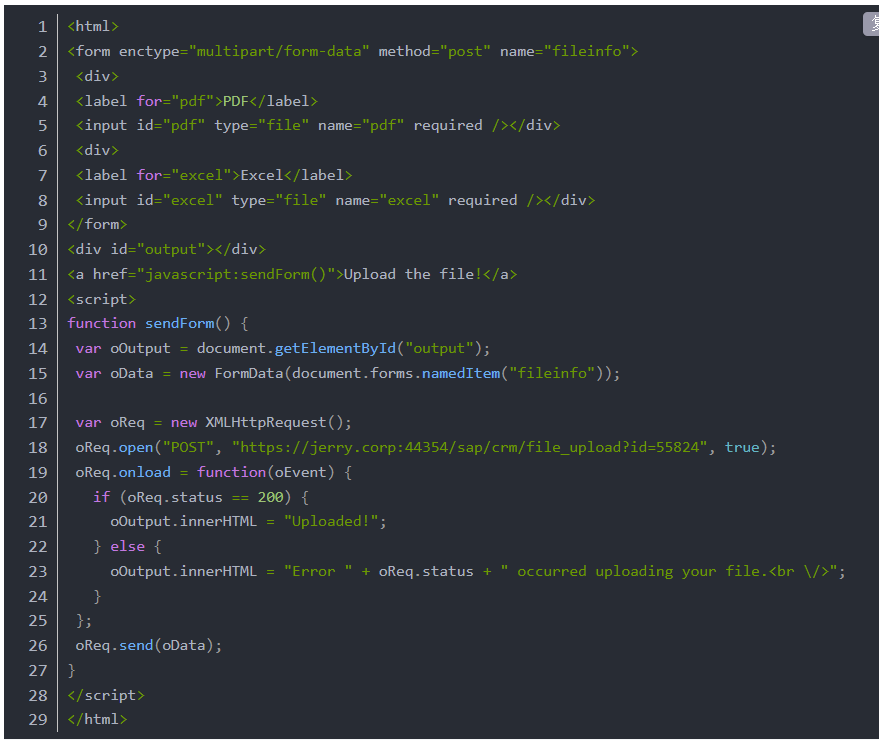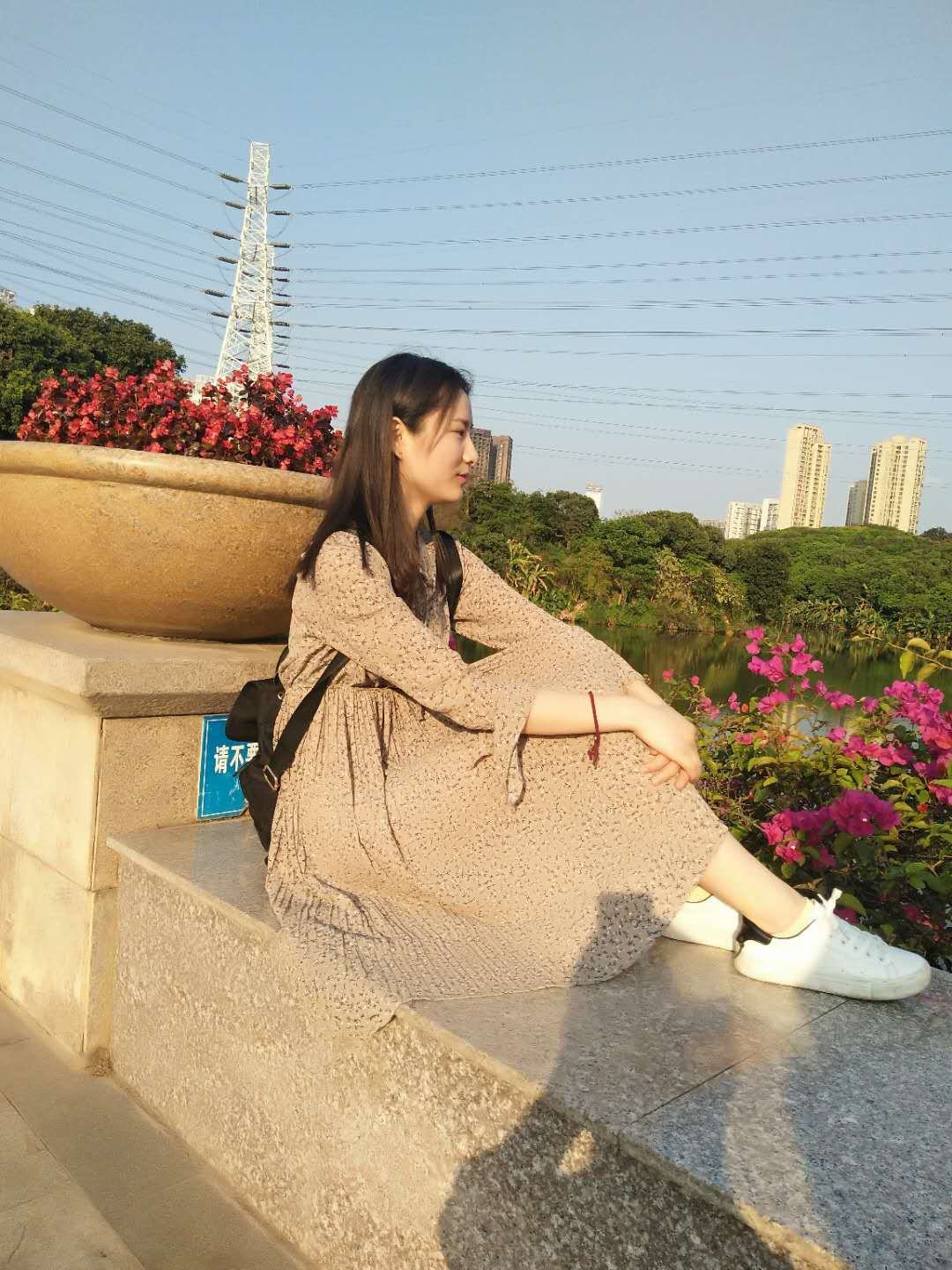使用SVG制作进度条之二

+关注继续查看简单的分析一下• 灰色表示进度条的底层（总共的长度）

• 红色表示进度条的进度 （已完成长度）

• 绿色表示起点 （跟随圆点）

• 水平进度条，灰色和红色是由<line>元素构建，绿色的由<circle>构建

• 圆形进度条由三个<circle>构建

[xml] view plain
1.

<svg width="200" height="200" viewBox="0 0 200 200">    <!-- 水平进度条 -->    <line x1="10" y1="10" x2="180" y2="10"fill="none" stroke-width="12" stroke="#666" stroke-linecap="round" />    <line x1="10" y1="10" x2="180" y2="10" fill="none" stroke-width="12" stroke="#FC4D04" stroke-dasharray="170" stroke-dashoffset="90" stroke-linecap="round" id="lineInner" />    <circle cx="10" cy="10" r="3" fill="none" stroke="green" stroke-width="6" />    <!-- 圆形进度条 -->    <circle cx="100" cy="120" r="74" fill="none" stroke="#666" stroke-width="12" />    <circle cx="100" cy="120" r="74"fill="none" stroke="#FC4D04" stroke-width="12" stroke-dasharray="465" stroke-dashoffset="400" stroke-linecap="round" />   <circle cx="100" cy="120" r="74" fill="none" stroke="green" stroke-width="12" stroke-dashoffset="464.5" stroke-dasharray="465" stroke-linecap="round" />
</svg>

document.querySelectorAll('line').getTotalLength() // => 170

[xml] view plain
1.

<circle cx="10" cy="10" r="3" fill="none" stroke="green" stroke-width="6" id="circle" style="transform: translateX(80px);"/>

document.querySelectorAll('circle').getTotalLength(); // =>464.2044677734375

Math.PI * 2 * 74// => 464.9557127312894rad = (Math.PI * deg) / 180deg = (rad * 180) / Math.PI65 / 74 * 180 / Math.PI = 50.32737389662636

添加动画效果

SVG中改变stroke-dashoffset的值，可以实现线条自画的效果。这并不是什么新东西，也不是复杂的东西。在我们今天的示例当中的关键点是怎么当绿色的点跟着移动。

[sql] view plain
1.

.getTotalLength() - stroke-dashoffset

(.getTotalLength() - stroke-dashoffset) / r; // => 弧度值

[sql] view plain
1.

(.getTotalLength() - stroke-dashoffset) / r * 180 / Math.PI; =>角度值

[xml] view plain
1.

<div id="app">    <svg width="200" height="200" viewBox="0 0 200 200">        <line x1="10" y1="10" x2="180" y2="10" fill="none" stroke-width="12" stroke="#666" stroke-linecap="round" />        <line x1="10" y1="10" x2="180" y2="10" fill="none" stroke-width="12" stroke="#FC4D04" stroke-dasharray="170" :stroke-dashoffset="dashOffsetLine" stroke-linecap="round" id="lineInner" />        <circle cx="10" cy="10" r="3" fill="none" stroke="green" stroke-width="6" id="circle" style="transform: translateX(80px);" id="circle"/>        <circle cx="100" cy="120" r="74" fill="none" stroke="#666" stroke-width="12" />        <circle cx="100" cy="120" r="74" fill="none" stroke="#FC4D04" stroke-width="12" stroke-dasharray="465" :stroke-dashoffset="dashOffsetCircle" stroke-linecap="round" />        <circle cx="100" cy="120"r="74" fill="none" stroke="green" stroke-width="12" stroke-dashoffset="464.5" stroke-dasharray="465" stroke-linecap="round" id="circleInner" style="transform:rotate(.8783783783783784rad); transform-origin: 100px 120px;"/>    </svg>    <div class="action">        <div class="line">            <label for="">line: stroke-dashoffset = "{{dashOffsetLine}}"</label>            <input type="range" name="points" min="0" value="{{dashOffsetLine}}" v-model="dashOffsetLine" max="170" step="1" @input="moveLine">        </div>        <div class="circle">            <label>circle:stroke-dashoffset = "{{dashOffsetCircle}}"</label>            <input type="range" name="points" min="0" value="{{dashOffsetCircle}}" v-model="dashOffsetCircle" max="465" step="1" @input="moveCircle">        </div>    </div>
</div>

[javascript] view plain
1.

let app = new Vue({    el: '#app',    data () {
return {            dashOffsetCircle: 400,            dashOffsetLine: 90,        }    },    methods: {        moveLine: function (e){            let circleLine = document.getElementById('circle')
let lineInnerLen = document.getElementById('lineInner').getTotalLength()            circleLine.style.transform="translateX(" + (lineInnerLen - e.target.value) + "px)"        },        moveCircle: function(e) {
let circleInner = document.getElementById('circleInner')
let circleInnerLen = Math.ceil(document.getElementById('circleInner').getTotalLength())
let arcLen = circleInnerLen - this.dashOffsetCircle

Vue代码写得比较拙逼，路过的大婶请多多指点。这个时候，你拖动input，改变其值时，就可以看到对应的效果。总结872 01065 09980 0background-image使用svg如何改变颜色
1362 013753 011875 010 0C/C++使用VOID指针保存结构体数据到二进制文件并且读取

866 07317 01116 0

20710

438

《2021云上架构与运维峰会演讲合集》

《零基础CSS入门教程》

《零基础HTML入门教程》